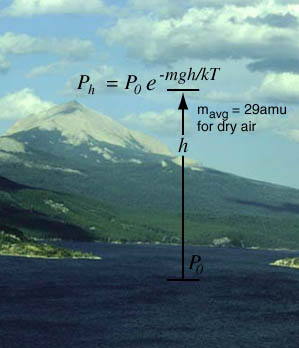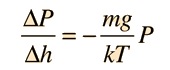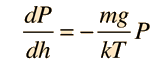# The Barometric FormulaIf the atmospheric pressure at ground level is P0 = mmHg = inHg = kPa and the temperature is uniform at K = °C then the pressure at an altitude of h = m = ft is Ph = mmHg = inHg = kPa This calculation uses m = amuM = kg/mol.

Note that the model calculation assumes a uniform temperature, and is therefore not a realistic model of the atmosphere. The temperature tends to decrease with height, so the model calculation will overestimate the pressure at a given height.

 Derivation of formula Pressure at selected heights Constituents of air
Index

Gas law concepts

Kinetic theory concepts

 HyperPhysics***** Thermodynamics R Nave
Go Back

# The Barometric FormulaStarting at some point in midair, the change in pressure associated with a small change in height can be found in terms of the weight of the air.The change in pressure depends on density, but ρ depends on the pressure as follows:The solution for the change from the ground
( P0) to height h ( Ph ) givesCalculation Details of solution

The development of the barometric formula makes use of a number of concepts from kinetic theory, such as the ideal gas law and the associated molecular constants. In the exponential, the two terms have the units of energy. The numerator mgh is gravitational potential energy and the term kT is thermal energy.

Index

Gas law concepts

Kinetic theory concepts

 HyperPhysics***** Thermodynamics R Nave
Go Back

# The Barometric FormulaHaving shown that the rate of change of pressure with height has the formit is necessary to take the limit as the change in height approaches zero, putting it in the form of a derivative.This type of equation can be solved for P by making a substitution of the typefitting the boundary conditions givesCalculation Form of solution
Index

Gas law concepts

Kinetic theory concepts

 HyperPhysics***** Thermodynamics R Nave
Go Back

# The Barometric FormulaThe equation for the variation of barometric pressure with height has the formwhich has the formal solutionSubstituting the solution givesSince this equation must be valid for all values of h, forcing the solution to fit the physical boundary conditions yields:Calculation Fitting boundary conditions
Index

Gas law concepts

Kinetic theory concepts

 HyperPhysics***** Thermodynamics R Nave
Go Back

# Atmospheric Pressure, Selected Heights

Compared to the standard Atmospheric pressure at sea level, 760 mmHg = 760 torr, the pressure at some heights above the earth are:

 Height Measured pressure Predicted pressure 30 km (19 mi) 9.5 torr 25 torr 60 km (37 mi) 0.21 torr .8 torr 90 km (56 mi) 0.0019 torr .03 torr

These pressures are considerably below those predicted by the barometric formula, which can be used to calculate variations in barometric pressure with height near the earth. (The predicted pressures above used temperature 300K and pressure 760 mmHg.) Though the pressure decreases rapidly, even at 200 km (124 mi) there is enough residual atmospheric pressure to gradually slow a satellite, limiting its lifetime.

Index

Gas law concepts

Kinetic theory concepts

 HyperPhysics***** Thermodynamics R Nave
Go Back

# Constituents of the Air

The constituents of dry air can be expressed as volume percentages, which will translate to the partial pressures out of the total atmospheric pressureSince a mole of any ideal gas occupies the same volume, it follows that the volume percentage is also the percentage by number. The average molecular mass can then be found by weighting the masses by their volume percentages above.

 Atmospheric pressure Barometric formula Respiration
Index

Gas law concepts

Kinetic theory concepts

 HyperPhysics***** Thermodynamics R Nave
Go Back